# Printable Version of Topic

### GBGL-HQ Forums _ General _ Finding power for any shot with KS Calc

#### Posted by: H3LLBane Jan 18 2007, 06:24 AM

Credits to creedo, i'm merely reposting his previous post.

QUOTE
When you change numbers in the formula, it updates automatically with your answer in the little window on the right.

What this formula does:
If you give it any desired angle, and any desired distance, it will spit out the exact power you need to make the shot. For example, let's say you have no idea what ice's 1 screen shot with angle 45 is.... just plug in the number for 1 screen distance, and the desired angle of 45, and it will spit out an answer of 2.24.

The formula that you copy and paste into KS Calc is:

See the number 800? That's the distance number, in pixels. One screen is 800, half screen is 400, 2 screens 1600, etc.

At the end of the formula is the number 70... that's the angle. You can change that to anything you want.

So after pasting the formula into KScalc, Just highlight the 800 and type in any distance you want, and highlight the 70 and type any angle that you want... and on the right will be the power level you need. Obviously if the power level shown is over 4 bars, you're trying an impossible shot (like try calculating power for 1 screen using angle 89 and it will tell you that you need 12 bars of power :P

This info is for a bot with Turtle's shot weight... but you can adjust it for other bots also.
To do that, change the bot's "gravity" and "shot speed" numbers. The gravity number is the "98" at the front of the formula. The shot speed is the "230" further on.

Shot weights and gravities are below

CODE

MOBILE SPEED GRAVITY
Armor 230 98
Mage 225 90
Nak Machine 205 98
Trico 215 98
Big Foot 210 98
Boomer 175 46
Raon Launcher 220 98
Lightning 235 90
J.D. 250 98
A. Sate 225 98
Ice 250 98
Turtle 230 98
Grub 250 98
Knight 250 90
Dragon 240 98
J.Frog 280 98
Kalsiddon 240 98

Now you can start making formula sheets of your own and enjoy fruit of your labour

#### Posted by: geLid Jan 18 2007, 08:14 AM

yay. im glad someone finally reposted this. thanks.

i had a question about it, now i won't have to figure it out the hard way...

What is "Rad" in the formula, and what exactly is it doing?

I'm assuming it's radians but I was trying to manipulate the formula and didn't know how I should approach it...

#### Posted by: VanPike Jan 18 2007, 09:13 AM

someone show me where i can get KS calc?

#### Posted by: ANBU Jan 18 2007, 10:55 AM

someone show me where i can get KS calc?

in the link in the very first post. ?

#### Posted by: CreeDo Jan 18 2007, 03:52 PM

if that link dies it's also at:
http://creedo.gbgl-hq.com/ks_calc.zip

"In calculus, angles must be represented in radians in trigonometric functions, to make identities and results as simple and natural as possible."

If you wanted to manipulate it, you can invert things the old fashioned way, algebraically.

divide both sides by 4:

square both sides to get rid of the sqrt:

multiply both sides by all the crap after 98*dist (remove the 1.00):

divide both sides by 98:

Bam, you got the distance.

Getting the angle is a little trickier. The function to algebraically invert the "sin" is arcsin, but kscalc doesn't take arcsin. MS Excel does though. They just write sqrt differently (with the t at the end). You can also write sin(big long formula)^-1 to get rid of the sin I think.

To get the angle from radians to degrees multiply by pi/180 ..about 0.01745

thanks creedo <3

#### Posted by: H3LLBane Jan 19 2007, 03:55 AM

LOL Creedo, i don't get a word of what you said, i learnt trigo and algerbra, but its the basic stuff lol.

#### Posted by: CreeDo Jan 19 2007, 04:14 PM

I didn't know this stuff either until I got into gunbound, it's kinda funny... I knew it'd never be useful for me, but I didn't guess it'd be useful for something useless like a video game.

#### Posted by: hellic Jan 19 2007, 04:16 PM

Now you have to use calculus to calculate tornado shots :O

#### Posted by: H3LLBane Jan 19 2007, 06:35 PM

Tornado shots are more of feel to me lol. If it enters the nado early, lower power, entering it late would mean more power, and thats with a high angle.

#### Posted by: mezz Feb 7 2007, 06:27 PM

Is this correct? (where the underscore stands for "in ______ units"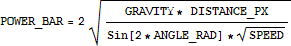Does anyone have mauve's original parametric shot formula?
It's not in the wayback machine and all the old forum archive is inaccessible to me.

#### Posted by: hellic Feb 7 2007, 06:30 PM

I derived this formula myself...and it's perfect (factors in wind too!).

airtime = 20*sqr(-15*(sin(angle)*xdist-cos(angle)*ydist))/(sqr(16(cos(angle)*sin(wind_degree)-sin(angle)*cos(wind_degree))*wind_strength-15*cos(angle)*mobile_gravity))

power=(2*ydist*800+4*mobile_gravity*airtime^2-4*wind_strength*(2/1.875))*sin(wind_degree)*airtime^2)/(2*airtime*shot_speed*sin(angle))

#### Posted by: mezz Feb 7 2007, 06:35 PM

Yeah hellic I got the same thing, actually. Not entirely sure how good it is to post it =/
Thanks for that though, mine is a bit too messy.

#### Posted by: hellic Feb 7 2007, 06:38 PM

Oh, trust me, it works fine ;D

I've had it for a while, but someone asked for a formula on the Softnyx Canada forum today, so I might as well paste it here too :X

#### Posted by: Rixxy Feb 7 2007, 06:39 PM

good luck squarerooting a negative number to get workable figures, faggot

#### Posted by: mezz Feb 7 2007, 06:40 PM

good luck squarerooting a negative number to get workable figures, faggot

lmfao. On closer look and as a clarification mine is similar, but not the same. XD

Rixxy's right hellic. Unless the rest of your thing comes out negative somehow.
If it does you can reverse the order and get rid of the negative

#### Posted by: hellic Feb 7 2007, 06:50 PM

Try the whole airtime formula with angle=70, both wind power and degree=0, y distance=0, and x distance=1/2 on Degree mode.

It works.

#### Posted by: mauve Feb 7 2007, 07:08 PM

Parametric:

CODE
x = cos(angle)*power*mobile_power_multiplier*time + (1/2)*horizontal_wind*time*time
y = sin(angle)*power*mobile_power_multiplier*time + (1/2)*(vertical_wind + mobile_gravity)*time*time

This is easily integrated via calculus, where the shot's speed at a given time is:

CODE
x' = cos(angle)*power*mobile_power_multiplier + horizontal_wind*time
y' = sin(angle)*power*mobile_power_multiplier + (vertical_wind + mobile_gravity)*time

To change from a parametric equation, simply substitute the other equation for time and integrate.

#### Posted by: mezz Feb 7 2007, 07:09 PM

It works, but you can replace
sqr(-15*(sin(angle)*xdist-cos(angle)*ydist))

with

sqr(15*(cos(angle)*ydist-sin(angle)*xdist)) I guess

EDIT:

Thanks mauve :D
Makes a lot more sense now, years later, because I've actually learned some calculus xD

EDIT:
My parametric graphs are coming out looking like glorified y=x^2 that just goes up forever.
Isn't it supposed to look like an upside down quadratic, going through the origin and comes back down to y=0 as T get larger?

I think I may have the wrong idea of mobile_power_multiplier... but I tried a few (positive) values and haven't gotten what I'm looking for

EDIT:

CODE
y = sin(angle)*power*mobile_power_multiplier*time - (1/2)*(vertical_wind + mobile_gravity)*time*time

This seems to give me what I'm looking for, but I'm not sure it's accurate. I made the 2nd part subtracted, instead of added which makes sense to me given that it's gravity.

#### Posted by: mauve Feb 7 2007, 08:19 PM

As I'm trying to avoid debugging some particularly painful shit right now, I'll fix that algorithm for you.

Since the goal here is to solve for power given a certain constant angle, distance, and height, then we get rid of the parametric formula and solve.

CODE
T = time
P = power
M = mobile power multiplier
G = gravity
H = horizontal wind
V = vertical wind

0 = (H/2)T^2 + cos(A)PM T - x
0 = ((V+G)/2)T^2 + sin(A)PM T - y

Apply quadratic formula to the second formula. The first will have H as a divisor, and will have
no quadratic solution unless horizontal wind exists.

CODE
T = -sin(A)PM (+/-) sqrt(sin^2(A)P^2M^2 + 2(V+G)y) / (V+G)

Of the two solutions for time, we only want the one with the negative solution. The positive result comes back with T = 0 as the solution for y = 0, and we don't want that.

CODE
T = -sin(A)PM - sqrt(sin^2(A)P^2M^2 + 2(V+G)y) / (V+G)

T^2 = (sin^2(A)P^2M^2 + sin(A)PMsqrt(sin^2(A)P^2M^2 + 2(V+G)y) + sin^2(A)P^2M^2 + 2(V+G)y)/((V+G)^2)
T^2 = (2sin^2(A)P^2M^2 + sin(A)PMsqrt(sin^2(A)P^2M^2 + 2(V+G)y) + 2(V+G)y)/((V+G)^2)

If that isn't making your eyes bleed yet, now we substitute.

CODE
0 = (H/2)(2sin^2(A)P^2M^2 + sin(A)PMsqrt(sin^2(A)P^2M^2 + 2(V+G)y) + 2(V+G)y)/((V+G)^2) + cos(A)PM(-sin(A)PM - sqrt(sin^2(A)P^2M^2 + 2(V+G)y) / (V+G)) - x

If y = 0, then the solution is substantially simplified.

CODE
0 = (3Hsin^2(A)P^2M^2/(2(V+G)^2) - (2cos(A)sin(A)P^2M^2 / (V+G)) - x

2cos(A)sin(A) = sin(2A)

0 = (3Hsin^2(A)P^2M^2/(2(V+G)^2) - (sin(2A)P^2M^2 / (V+G)) - x

Solve for P.

CODE
P^2( (3Hsin^2(A)M^2/(2(V+G)^2) - (sin(2A)M^2 / (V+G)) ) = x
P^2( (3Hsin^2(A)M^2/(2(V+G)^2) - (2(V+G)sin(2A)M^2 / 2(V+G)^2) ) = x
P^2( (3Hsin^2(A)M^2 - 2(V+G)sin(2A)M^2) / 2(V+G)^2) = x

P = sqrt( 2 x (V+G)^2 / (3Hsin^2(A)M^2 - 2(V+G)sin(2A)M^2)) [edit:typed this one wrong before]

If V and H = 0, then:

CODE
P = sqrt( x (G^2) / -Gsin(2A)M^2 )
P = sqrt( x G / -sin(2A)M^2 )

Does this look familiar? Because it should. Why is sin(2A) negative, you might be wondering? Because angle, by default, goes downwards. Just negate your angle and you get the correct solution.

CODE
P = sqrt( x G / -sin(-2A)M^2 )
P = sqrt( x G / sin(2A)M^2 )

I might have made a mistake somewhere, it's rather hairy looking. Solving the remaining problems, including the state of y =/= 0 is left as a problem to the rest of you.

#### Posted by: mezz Feb 7 2007, 08:56 PM

LMFAO.
I included Y by using a symbolic Solve[ ] function.

4 answers, all over a page long. O.o

Trying a reduce function... I have the odd feeling this is either going to crash my computer or just not work.

#### Posted by: mauve Feb 7 2007, 09:05 PM

Sometimes, the best results are done with a pencil and paper.

#### Posted by: mezz Feb 7 2007, 09:17 PM

It's down to 4 lines now, using the most basic simplification algorithm is has.
Using the full one now... zzz.
Hmm came up with something pretty long, but reasonable looking.

Luckily the 4 answers are just + or - versions of each other for the different square roots. I just need to plug some stuff in and see which are the one(s) I want.

EDIT:
I got
the same P = with those assumptions

As well as: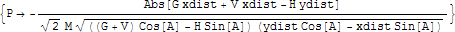#### Posted by: mauve Feb 8 2007, 12:57 AM

All that's left is to find the values to plug into it, huh? Well, good luck with that. I wonder how it derived that particular answer. The absolute part implies that it's getting rid of the root somehow, but damned if i know how.

H and V are just the individual components of the wind. Probably want to replace them with cos/sin of the wind angle, times wind power. Possibly times mobile wind resistance, too?

#### Posted by: mezz Feb 8 2007, 01:06 AM

It ended up with Sqrt(( with every value inside squared. I defined them as real numbers so it converted to an Abs sign.
I'm going to check it by hand tomorrow to make sure it's right though. Took me a while to figure out the program's syntax and abilities, but learning Mathematica and the calculator are my main goals from doing this anyway.

I actually got a positive version as well. I will need to make sure I eliminate the correct one.

#### Posted by: CreeDo Feb 8 2007, 04:22 PM

I missed something... M = Mobile power multiplier... what's that?
Some shorthand of the shot weight/speed?

If there isn't a shorthand for each bot's shot weight/speed, could one be made to make a formula shorter? Since each bot's combination of the two is unique?

#### Posted by: mauve Feb 8 2007, 04:45 PM

M would be the shot speed referred to in the original post.

G would be the gravity, or weight, of the shot.

And I don't see how you would eliminate those constants to simplify further.

#### Posted by: mezz Feb 8 2007, 07:28 PM

You can just write the formula "for turtle only" kind of thing I guess.

Assuming G=98 and M=230, wind=0

CODE
Power = ((7Sqrt(2))/115) * Sqrt(X_Dist_PX*Csc(ANGLE))

And ((7Sqrt(2))/115) = 0.08608256467...

But I doubt anyone can do csc in their head.

#### Posted by: CreeDo Feb 8 2007, 07:34 PM

yeah, mezz gets what I mean.

Write the formula as it would appear if speed/gravity were known and fixed. Reduce it to the simplest variables:
Distance/Vertical Distance/Angle/Power/Wind/Wind Angle/

All other stuff could be presolved and then made into a slightly easier formula. Prolly this is spoonfeeding a bit much but I like tidy things.

Then offer a different shortened version with those same variables for each bot.

#### Posted by: mezz Feb 8 2007, 07:38 PM

I'm working on it :D
I have lots of memory on my calculator, but very little power so I am trying to get a bunch of reduced formulas.

Quick noob check to make sure I don't screw something fundamental up:

W_Power*Sin(Wind_Angle)=Horizontal Wind
W_Power*Cos(Wind_Angle)=Vertical Wind

Right?
EDIT: never mind, duhhhh.

I haven't used trig in a while :Z

#### Posted by: Mammophobia Feb 9 2007, 05:20 PM

Well, seeing as how I have a Ti-83, all I basically did was make a Program that had the formula while inputting X,Y,Z,(Theta) values.

I use the formula above for power, and a few windcharts and voila, external aimbot =]

X= Distance (Pixels)
Y= Angle of Shot
Z= Wind Power
(Theta)= Wind Angle

#### Posted by: hellic Feb 9 2007, 05:54 PM

Well, seeing as how I have a Ti-83, all I basically did was make a Program that had the formula while inputting X,Y,Z,(Theta) values.

I use the formula above for power, and a few windcharts and voila, external aimbot =]

X= Distance (Pixels)
Y= Angle of Shot
Z= Wind Power
(Theta)= Wind Angle
Why do you still need windcharts? This formula accounts for wind, I think.

#### Posted by: Mammophobia Feb 9 2007, 06:14 PM

Why do you still need windcharts? This formula accounts for wind, I think.

Well, I just took the original equation (The one posted, plugged into the Calc) and just sort of made my own system of equations from there.

I think most of the posts here are to simplify equations with wind factored into them.

#### Posted by: Angeluz Jun 7 2007, 01:04 PM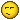, dont work me,i try with,

xdist = 26
wind = 0
angle = 89

in your formule i get -0.224515467254494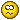, and the true result is 2.25

what happend..???
P := (-1) *
Abs( G * xDist + V * xDist + H * yDist ) /
(
Sqrt(2) * M * Sqrt
(
Abs( ((G+V)*Cos(A) - H*Sin(A)) * (yDist*Cos(A) - xDist*Sin(A)) )
)
);

#### Posted by: Casarino Jan 6 2009, 10:49 AM

i got a question if i wanna find out some 0 angle powers for example nak 70� i tip in my ks calc 4*Sqrt((98*800*1.00)/(4*Sqr(205)*Sin(Rad(2*70)))) so i get power 3.4072218... but creedos 70� 1 screen power is 3.36 where is my mistake? T_T

#### Posted by: PUTOOOOO Jan 6 2009, 06:33 PM

wrong mass or gravity

#### Posted by: Casarino Jan 7 2009, 06:46 AM

where i can get right gravity and mass? the turtle mass and gravity is exact like creedos info

#### Posted by: Htd Jan 7 2009, 03:48 PM

where i can get right gravity and mass? the turtle mass and gravity is exact like creedos info

Pm a physics user. Is turtle all you need? I'll ask my buddy if he knows.

#### Posted by: Casarino Jan 7 2009, 03:56 PM

uhm i would need for all bots if it is possible. turtle shotspeed and gravity looks okay but other like nak or ice not :/ would be great if u ask ty

#### Posted by: Casarino Jan 11 2009, 07:35 AM

pls can any one help me with that pm would be nice too :/

#### Posted by: ClosetMonkey Apr 18 2009, 06:26 PM

Hi everyone,

I'd like to start of by saying that english isn't my main language, so I'm sorry for any incomprehensible stuff.

Here we go,

So, how do you ajust for wind from the wind's vertical and horizontal forces for timed shots?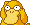The thing is, I searched for some physics equations and stuff and managed to made my own system of equations for turtle's SS and fork shot (inputing xdistance and ydistance), but I can only get accurate results for 0 wind shots. (From there I've been ajusting using Yoyobuae's aim chart)

QUOTE
Wh - wind's horizontal vector
Wv - the vertical one

x = Power*Constant*Airtime*Cos(Angle) + .5*Wh*Airtime^2

y = Power*Constant*Airtime*Sin(Angle) + .5(Wv + Gravity)*Airtime^2

This is obviously (duh) not the final thing but the thing is, I feel like (the bold part):

QUOTE
x = Power*Constant*Airtime*Cos(Angle) + .5*Wh*Airtime^2

y = Power*Constant*Airtime*Sin(Angle) + .5(Wv + Gravity)*Airtime^2

...should have something concerning the wind's effect.

The only "solution" I've found so far is creating constant for the wind's effect (like 1, for example) and then comparing the results (obviously wrong) with the ones i got from Yoyobuae's and making some kind of windchart crap, but that is out of the question, cause I'd have to make one for every angle and wind angle to make it accurate.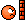So, does anyone know how to solve this problem?
(It should be possible, since aimbots have fixed-time thingy)

(I guess I came out of the closet)

EDIT: Oh, I forgot to mention, the constants i used for both Gravity and MobileConstant were found by attempt and they're not the same (neither Gravity, nor MobileConstant) for Timebomb and Fork shot (I used 1.83 secs, in case you want to know).

#### Posted by: v1d4l0k4 Apr 22 2009, 12:52 PM

Does anyone have the speed and gravity of the new mobiles (BlueWhale, Frank, Maya, Phoenix, Tiburon, Wolf)?

PS: What the name of this formula?

Thanks,
v1d4l0k4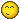#### Posted by: PUTOOOOO Apr 22 2009, 06:05 PM

Does anyone have the speed and gravity of the new mobiles (BlueWhale, Frank, Maya, Phoenix, Tiburon, Wolf)?

PS: What the name of this formula?

Thanks,
v1d4l0k4I call this formula Yukon Jack!

Delta Method

#### Posted by: PUTOOOOO Apr 22 2009, 08:04 PM

NO, IT'S THE YUKON JACK

#### Posted by: ClosetMonkey Apr 24 2009, 03:01 PM

Hey guys,

So, I had an idea concerning my post (didn't quote it cause it's too big ang ugly)

According to Yoyobuae's chart (the 2.15secs one for turtle), 20 wind is like 200 pixels, so maybe there is a way to ajust it without having to find any constant for the wind's effect. You got the point, unless you're a complete mongoloid, which you certainly are not!

/////

I finally had time to work on it, and it works, just to let you know. For fork I used like 200/29 or 26 ionno what works better.

But there is a problem, when wind is around angle 56-68 it's clear that it won't reach, so I usually have to reduce 1 to 3 angles.